Ray Tracing

Let's catch some rays

Introduction

Tracing rays of light from an object through a lens or off a mirror can help you to locate an image.

Material

• Paper
• ruler
• pencil

Assembly

To Do and Notice

Lenses
Converging lenses

Let's begin with a common lens that is thicker in the middle than at the edges.
Most lenses are circular, the axis of the lens is the first line to remember. If you consider the lens as a wheel the axis of the lens will be an axle through the wheel. We will consider all lenses to be thin lenses. Real lenses light bends at both the front and the rear surface, however if the lens is thin then you can consider the light to bend at a line through the center of the lens that is perpendicular to the axis.

Lenses are characterized by focal points.

The first important rule of ray tracing is:

Light rays parallel to the axis will all bend so as to pass through a focal point of the lens.

To find the focal point of a converging lens start with a distant light source. Find the place where the image made by the lens is the most pointlike. This will be the focal point. The focal length is the distance from the center of the lens to this point. By the way, distant means ten or more focal lengths away, so after you find the focal point check to see if the source is truly far away.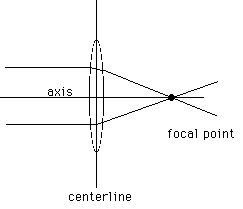A lens has an axis and a centerline. In a real converging lens light bends at both surfaces as shown by the upper ray, in the thin lenses used in ray tracing we model light bending once at the centerline as shown by the lower ray. Light rays parallel to the axis all cross at a focal point of the lens.

One of the important laws of optics is the law of reversibility, if you reverse a light ray and send it back along its path it will exactly reverse its entire path. This means that light which passes through a focal point and hits a converging lens will exit the lens parallel to the axis.

Light rays spread out from each and every point on an object in all directions, like the spines of a sea urchin. A converging lens will bend these lines of light so that if the object is farther from the lens than a focal point, the rays of light will pass through one point on the other side of the lens. This point is on the image of the object. There are three rays which are usually used to find where the image of an object is located.

1. The parallel ray is a ray from the object parallel to the axis of the lens. It goes through the focal point on the side of the lens opposite the object.

2. The chief ray passes through the center of the lens, for a thin lens it is a single straight line, it is not bent by the lens.

3. The focal ray goes through the focal point on the same side of the lens as the object. The focal ray exits parallel to the lens by the law of reversibility.

The image made by a converging lens will appear to be at the point where these rays cross.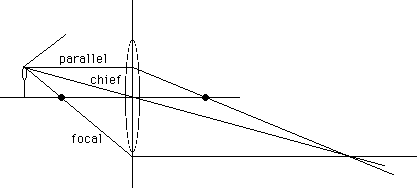Light rays spread from a point on an object and bend at a lens to form and image point where the rays all cross. Three rays are easy to trace. The parallel ray, the chief ray through the center of the lens, and the focal ray through the near focal point. Notice that rays can be used to locate images even if they do not hit the lens, the rays that do hit the lens will make an image at that spot.

As shown here. an object outside the focal point of a converging lens makes an image outside the focal point on the opposite side of the lens. The other case of the object inside the focal point is also worth drawing.

When light actually passes through the location of the image, the image is called a real image. A real image can be photographed by placing film at the location of the image.

Diverging lens

A diverging lens bends light which is parallel to the axis away from the axis.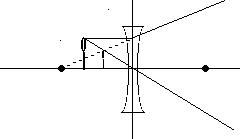Light from an object is bent away from the axis of a diverging lens, creating a virtual image.

With all lenses always begin by drawing the chief ray.

Then with a diverging lens draw the parallel ray which, after passing through the lens, diverges as if it comes from the focal point.

The image is located at the intersection of these rays.

Real light paths are shown as solid lines. The real light paths never cross at the image location, therefore this is a virtual image.

Mirrors

For a thin mirror we trace the rays as they bounce off an imaginary flat plate tangent to the mirror at its axis. The main difference from lenses is the chief ray which bounces off the center of the mirror as if it were a flat plate. Notice the parallel ray, parallel to the axis, and the focal ray, through the focal point.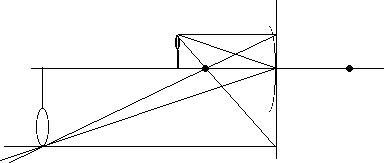A converging mirror makes an inverted real image of an object outside the focal point.

The light rays actually cross in this case so they make a real image.

Diverging Mirror

The chief ray behaves the same for this mirror as for the converging mirror. The parallel ray bounces off the mirror and diverges as if it came from the far focal point. The rays appear to cross behind the mirror, making a virtual image.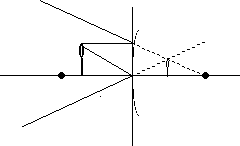A convex or diverging mirror makes virtual images behind the mirror.

What’s Going On?

Here are some practice drawings on which to draw rays.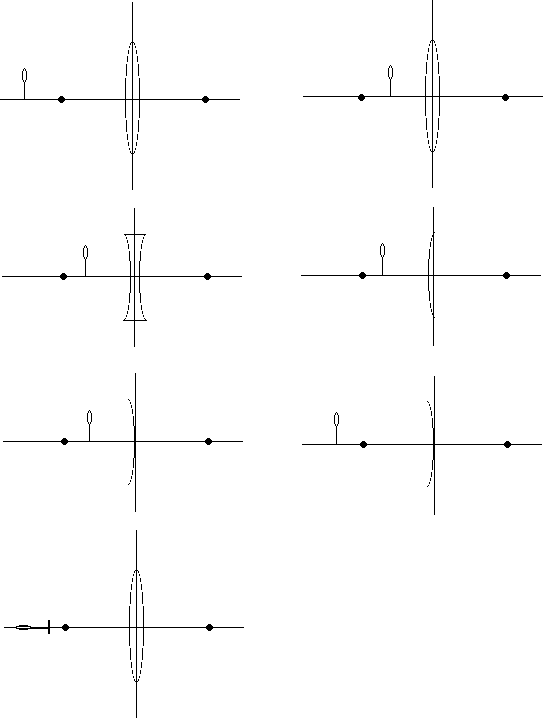Etc

If you shine a pointer laser through a lens or onto the mirror the beam from the laser will closely follow the path of the ray predicted by ray tracing.